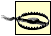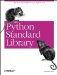# The random Module

"Anyone who considers arithmetical methods of producing random digits is, of course, in a state of sin."

John von Neumann, 1951

The random module contains a number of random number generators.

The basic random number generator (after an algorithm by Wichmann and Hill, 1982) can be accessed in several ways, as Example 2-29 shows.

Example 2-29. Using the random Module to Get Random Numbers

```File: random-example-1.py

import random

for i in range(5):

# random float: 0.0 <= number < 1.0
print random.random(),

# random float: 10 <= number < 20
print random.uniform(10, 20),

# random integer: 100 <= number <= 1000
print random.randint(100, 1000),

# random integer: even numbers in 100 <= number < 1000
print random.randrange(100, 1000, 2)

0.946842713956 19.5910069381 709 172
0.573613195398 16.2758417025 407 120
0.363241598013 16.8079747714 916 580
0.602115173978 18.386796935 531 774
0.526767588533 18.0783794596 223 344```

Note that the randint function can return the upper limit, while the other functions always return values smaller than the upper limit.

Example 2-30 shows how the choice function picks a random item from a sequence. It can be used with lists, tuples, or any other sequence (provided it can be accessed in random order, of course).

Example 2-30. Using the random Module for Random Items from a Sequence

```File: random-example-2.py

import random

# random choice from a list
for i in range(5):
print random.choice([1, 2, 3, 5, 9])

2
3
1
9
1```

In 2.0 and later, the shuffle function can be used to shuffle the contents of a list (that is, generate a random permutation of a list in-place). Example 2-31 also shows how to implement that function under 1.5.2 and earlier.

Example 2-31. Using the random Module to Shuffle a Deck of Cards

```File: random-example-4.py

import random

try:
# available in 2.0 and later
shuffle = random.shuffle
except AttributeError:
def shuffle(x):
for i in xrange(len(x)-1, 0, -1):
# pick an element in x[:i+1] with which to exchange x[i]
j = int(random.random() * (i+1))
x[i], x[j] = x[j], x[i]

cards = range(52)

shuffle(cards)

myhand = cards[:5]

print myhand

[4, 8, 40, 12, 30]```

The random module also contains random generators with non-uniform distribution. Example 2-32 uses the gauss function to generate random numbers with a gaussian distribution.

Example 2-32. Using the random Module for Gaussian Random Numbers

```File: random-example-3.py

import random

histogram =  * 20

# calculate histogram for gaussian
# noise, using average=5, stddev=1
for i in range(1000):
i = int(random.gauss(5, 1) * 2)
histogram[i] = histogram[i] + 1

# print the histogram
m = max(histogram)
for v in histogram:
print "*" * (v * 50 / m)

****
**********
*************************
***********************************
************************************************
**************************************************
*************************************
***************************
*************
***
*```The random-number generators provided in the standard library are pseudo-random generators. While this might be good enough for many purposesincluding simulations, numerical analysis, and gamesit's definitely not good enough for cryptographic use.Python Standard Library (Nutshell Handbooks) with
ISBN: 0596000960
EAN: 2147483647
Year: 2000
Pages: 252
Authors: Fredrik Lundh